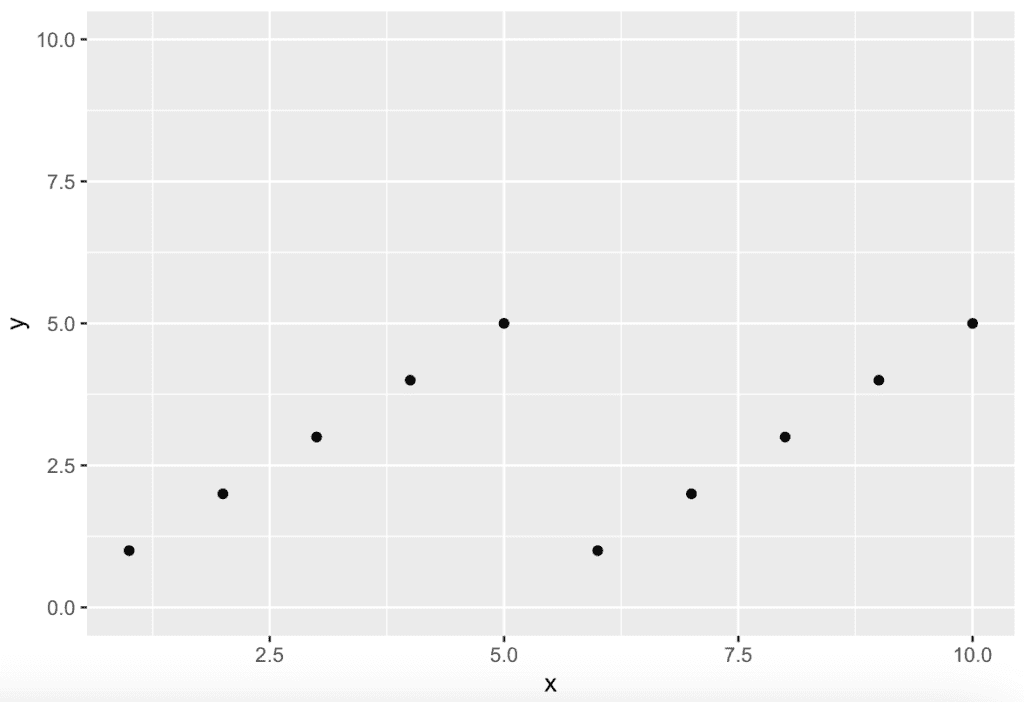# How to Fix R Error: discrete value supplied to continuous scale

The error: discrete value supplied to continuous scale typically occurs in R when you use erroneous use of the scale function along with ggplot().

``````library(ggplot2)

df <- data.frame(x = 1:10, y = c("bmw", "audi", "mercedez", "ferrari", "jaguar"))

ggplot(df, aes(x, y)) + geom_point() + scale_y_continuous(limits = c(0, 10))``````

Output

``Error: Discrete value supplied to continuous scale``

If you have not installed the ggplot2, you can install it in RStudio and by command.

``install.packages("ggplot2")``

After installing, you need to import the library in your R Script.

``library(ggplot2)``

The code you provided is causing the same error, “discrete value supplied to continuous scale” because you’re trying to plot a categorical variable “y” on a continuous scale.

Even if you set the limits of the y-axis to a range of continuous values, it doesn’t change the fact that the variable “y” is categorical.

## How to fix error: discrete value supplied to continuous scale in R

Here are two solutions to fix the error: discrete value supplied to continuous scale in R.

1. Use the numeric vector.
2. Map the categorical variable to a discrete scale, such as a factor, using the “as.factor()” function.

### Solution 1: Use the numeric vector

``````library(ggplot2)

df <- data.frame(x = 1:10, y = c(1:5))

ggplot(df, aes(x, y)) + geom_point()
+ scale_y_continuous(limits = c(0, 10))``````

Output### Solution 2: Use the as.factor() function

To fix the error, another approach is to use the as.factor() function and convert the variable “y” to a factor before plotting it.

The as.factor() is a built-in R function that converts an object like a vector or a data frame column from numeric to factor.

``````ggplot(df, aes(x, as.factor(y)))
+ geom_point()
+ scale_y_continuous(limits = c(0, 10))``````

It will probably resolve the error you are facing!

That’s it.